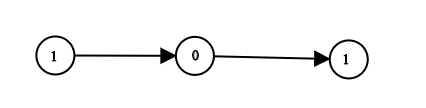# GeetCode Hub

Given `head` which is a reference node to a singly-linked list. The value of each node in the linked list is either 0 or 1. The linked list holds the binary representation of a number.

Return the decimal value of the number in the linked list.

Example 1:```Input: head = [1,0,1]
Output: 5
Explanation: (101) in base 2 = (5) in base 10
```

Example 2:

```Input: head = 
Output: 0
```

Example 3:

```Input: head = 
Output: 1
```

Example 4:

```Input: head = [1,0,0,1,0,0,1,1,1,0,0,0,0,0,0]
Output: 18880
```

Example 5:

```Input: head = [0,0]
Output: 0
```

Constraints:

• The Linked List is not empty.
• Number of nodes will not exceed `30`.
• Each node's value is either `0` or `1`.

/** * Definition for singly-linked list. * public class ListNode { * int val; * ListNode next; * ListNode() {} * ListNode(int val) { this.val = val; } * ListNode(int val, ListNode next) { this.val = val; this.next = next; } * } */ class Solution { public int getDecimalValue(ListNode head) { } }